## Friday, January 20, 2017

### Employee Scheduling I: Matlab vs GAMS

In (1) a small employee scheduling problem is modeled as a simple MIP model using MATLAB. Basically from the data on employee availability, we need to form all possible shifts. Even for small data sets that can become a large number. Having all these possible shifts we introduce a binary variable:

 $x_s = \begin{cases}1 & \text{if shift s is selected}\\ 0 & \text{otherwise} \end{cases}$

We have some requirements with respect to staffing levels, and an employee can only do one shift in the 24 hours we are look at. The standard model for this is:

 \begin{align}\min\>&\sum_s c_s x_s\\ &\sum_{s|E(e,s)} x_s \le 1\>\forall e\\ &\sum_{s|S(s,t)} x_s \ge R_t \> \forall t\\ &x_s \in\{0,1\}\end{align}

The first constraint says each employee can perform at most one shift. The second constraint makes sure that we have enough shifts selected such that we meet staffing requirements. The objective minimizes the cost associated with the selected shifts. This is a very simple model, so actually the main issues are with data manipulation: we need to form a collection of all possible shifts.

#### Data

We reproduce here the data from (1):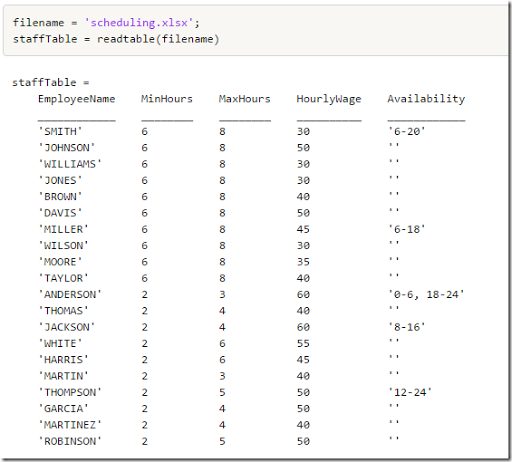Data entry using Excel is always very convenient. Most users know and have access to Excel. Notice that the last column  (Availability) is a string, which needs to be parsed inside the application.  Matlab has sscanf, GAMS has no similar facilities. If you want to directly read this into GAMS you could use 4 columns, say Start1, End1, Start2, End2 with integer values.

The staffing requirements are: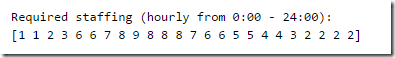#### Matlab code

The Matlab code from (1) and (2) combines generating the shifts and producing the LP matrix. This makes it more difficult to understand the model: it is hidden inside the code. I quickly scanned the code, but decided not to translate this into GAMS but rather start from scratch. Translating code line-by-line is often not as useful.

The blog post (1) displays the non-zero pattern of the LP matrix. In my opinion, in a GAMS environment such a picture is not very useful.

#### GAMS code: generating shifts

The GAMS code I wrote separates generation of shifts from the model equations. As it turns out the first task is not completely trivial.

The first thing I did was to generate a set canwork(e,t) containing whether a time slot is allowed by an employee: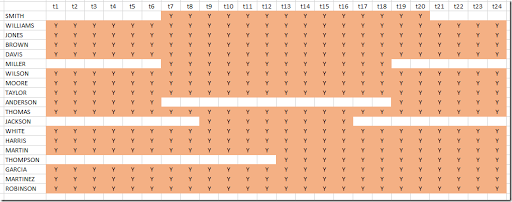This was produced from the rules:

 canwork(e,t)$(data(e,'start1')=0 and data(e,'end1')=0) = yes;canwork(e,t)$(ord(t)>data(e,'start1') and ord(t)<=data(e,'end1')) = yes;canwork(e,t)$(ord(t)>data(e,'start2') and ord(t)<=data(e,'end2')) = yes; The next thing I did was to generate a parameter maxstart(e,t) indicating how long the maximum shift can be starting in period t. This looks something like: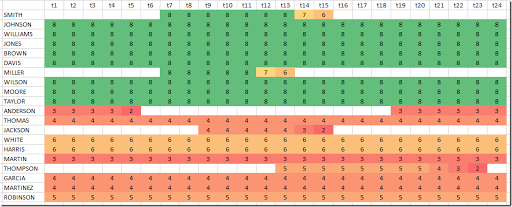It is not simple to compute. Note that near the end of the day we still can start a long shift: shifts wrap from t24 to t1. Here is my code:  set back(t,tt) 'used to loop backwards over time';back(t,tt)$(ord(tt)+ord(t)=card(t)+1) = yes;* when looping over this set tt runs backwardsparameters   count0(e,t)    'max length of remaining part of shift in t1'   maxstart(e,t)  'max length of shift starting in t';** First calculate maximum length of remaining part of shift in t1* This is used when wrapping from t24 to t1.* Notes:*   We scan backwards.*   count0(e,t) is indexed by t, but we only use t=t1.*count0(e,t) = 0;loop(back(t,tt),   count0(e,'t1')$canwork(e,tt) = min(count0(e,'t1')+1,maxlen(e)); count0(e,'t1')$(not canwork(e,tt)) = 0;);** Now calculate maximum length of shift starting in t*maxstart(e,t) = 0;loop(back(t,tt),   maxstart(e,tt)$canwork(e,tt) = min(maxstart(e,tt+1)+1+count0(e,t),maxlen(e)););maxstart(e,tt)$(maxstart(e,tt)

From this we can compute the following:

1. The set shifts(s,t) with a shift id s and hour t.
2. A mapping set esmap(e,s), that maps between employee e and shift s.
3. A subset s of used shifts.

The data structure shifts looks like: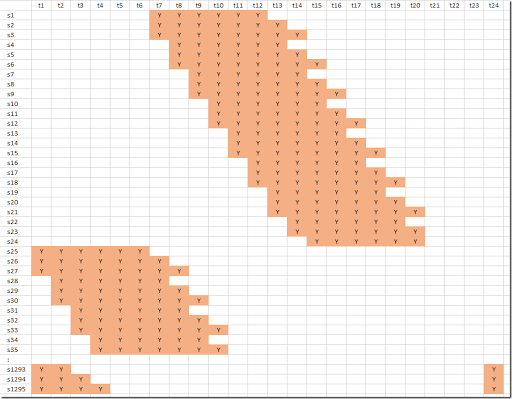We can see here just a part of the shifts. There are 1295 of them. Near the bottom we see a few shifts wrapping from t24 to t1.

Shifts s1 through s24 belongs to employee Smith; Johnson owns shifts s25-s96. This correspondence is stored in the mapping set esmap(e,s). The code to generate this is:

 sets  s0 'shifts'  /s1*s10000/  s(s0) 'used shifts'  k(s0) /s1/;scalar i;loop((e,t)$maxstart(e,t), for(i = minlen(e) to maxstart(e,t), shifts(k,tt)$(ord(tt)>=ord(t) and ord(tt)=1 and ord(tt)

Boy this was not straightforward, and the code is not very intuitive. But we managed. Probably using a standard programming language this shift generation step may be easier.

A very different technique is to use an optimization model in combination with the Cplex solution pool technology to generate all possible shifts. In (4) this approach is applied to this problem.

#### GAMS code: MIP model

Finally we need to implement optimization model. This is actually very easy now:

 parameter shiftlen(s0) 'number of hours in a shift';shiftlen(s) = sum(shifts(s,t),1);binary variable x(s0);variable cost;equations   oneShift(e)     'each employee can do at most one shift'   coverPeriod(t)  'cover each period'   calcCost        'calculate total cost';oneShift(e)..   sum(esmap(e,s), x(s)) =l= 1;coverPeriod(t)..   sum(shifts(s,t), x(s)) =g= requirements(t);calcCost..  cost =e= sum(esmap(e,s),  wage(e)*shiftlen(s)*x(s));model m /all/;option optcr=0;solve m minimizing cost using mip;

This model follows very closely the mathematical model presented at the beginning of this post. This model solves very fast. Cplex can find the optimal solution (proving optimality) without doing any branching. The problem with this formulation is that it can generate a ton of possible shifts, but an advantage is that it often solves very fast. The results are: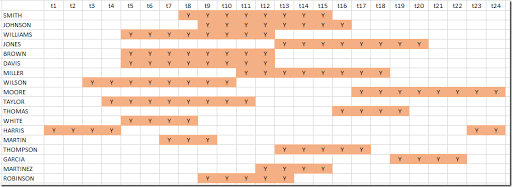This is different from what is shown in (1) but with the same objective: the cost is \$4670. As in (1) there is no slack: we precisely match the staffing requirements: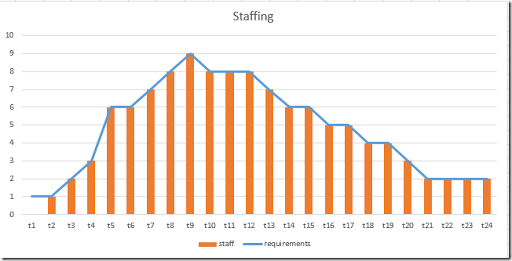#### References

1. Loren Shure, Generating an Optimal Employee Work Schedule Using Integer Linear Programming, January 6, 2016, http://blogs.mathworks.com/loren/2016/01/06/generating-an-optimal-employee-work-schedule-using-integer-linear-programming/
2. http://blogs.mathworks.com/images/loren/2016/makeMILPMatrices.m contains the matrix generation code.
3. Employee Scheduling II : Column Generation, http://yetanothermathprogrammingconsultant.blogspot.com/2017/01/employee-scheduling-ii-column-generation.html uses a Column Generation approach to solve this problem.
4. Employee Scheduling III : generating all shifts using the Cplex solution pool, http://yetanothermathprogrammingconsultant.blogspot.com/2017/01/employee-scheduling-iii-generating-all.html uses the Cplex Solution Pool technology to generate all possible shifts. This is an alternative approach to the method shown in this post.
5. Employee Scheduling IV: direct optimization, http://yetanothermathprogrammingconsultant.blogspot.com/2017/03/employee-scheduling-iv-direct.html